﻿ houghton biology site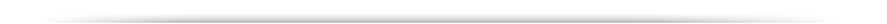To Recap Aspects of Mendelian Genetics that we went over the other day.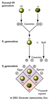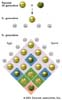...test cross?

Ratios: 3:1 and 1:1 (test cross) for monihybrid crosses and 9:3:3:1 for dihybrid crosses.

Extension of Mendelian Genetics:-

 Incomplete dominance: eg. Four o' clocks, carnations. BLENDING 1:2:1 Codominance: eg. M and N blood groups on chromosome 4 having specific antigens M and N 1:2:1 but where the heterozygotes (MN) gives rise to a distinct phenotype Multiple alleles ABO blood types, A and B are dominant to O , but A and B codominant to each other Lethal alleles eg. Yellow colouration in mice fur. 2 : 1 . Pleiotropy(?) Several genes/same character Coat colour in mammals, eg. mice A (agouti), B (black/brown), C, (colour) D (intensity), S. (distribution) genes Epistasis essentially "eliminates" or masks phenotypic expression of other genes, eg. Labrador dogs fur colour, albinoism in mice Complementary Genes eg. Pea plants, purple colouration. 9 : 7 Duplicate gene activity eg. Shepherd's purse, Round over narrow fruits, where both A 1 - and A 2 - can cause heart shape 15 : 1 Recessive suppression eg. Case of purple eye colour in the fruit fly. 13 : 3

Discovery of Linkage: T. S. Morgan (1909) working on non-autosomal and sex chromosome -linked genes of Drosophila melanogaster -the commmon fruit fly, and implications in understanding X linkage in humans.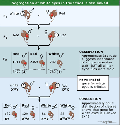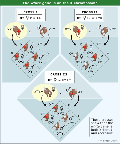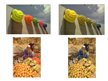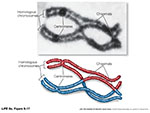While the X and Y  chromosomes normally behave according to the laws of meiotic cell division (and thus according to Mendelian genetics) the ability to "visualize" through genetic crosses the presence of each of the alleles that is located on either of the X-chromosomes of a mother directly challenges the ubiquity of Mendel's 2nd law).

Remember, that... to equate Mendel with meiosis we had to invoke the role of chiasmata occuring between two gene loci on adjacent chromatids in paired chromosomes during prophase I of meiosis.   This CANNOT occur for X and Y chromosomes,

But what about ALL the genes on the remainder of the non sex chromosomes or "autosomal" chromosomes.

I glibbly stated th other day that, if the probability of a chiasma forming between two genes is "one" (i.e. a certainty), then the assortment of alleles would, in essence, be the same as if they were on separate chromosomes (i.e. random).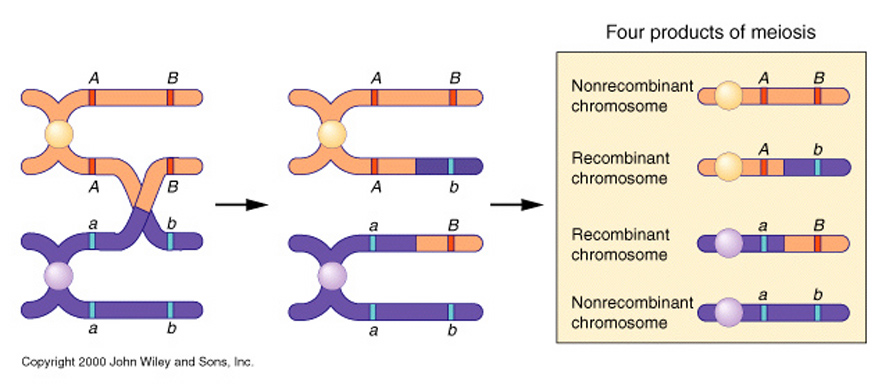But Morgan went further. He analyzed the likelihood of the hereditary outcomes of TWO genes "white eye" and "miniature wing". which he knew (from previous studies) were on the same X chromosome,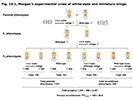Morgan then went on to analyze other genes and demonstrated that some genes -other than the ones that he had tracked to the X chromosome- also failed to behave according to Mendelian expectations, and appeared to retain some of the gene association traits that were present to one or the other of the parents.

Two such traits (not on the X chromosome): purple eye colour (pr, purple and pr+, red) and vestigial wing (vg, vestigial and vg+ normal).

cross       pr+ pr+ and vg+ vg+  x   pr pr and vg vg

giving an -> F1 gen. of      pr+ pr / vg+ vg.

He then test crossed the females of these flies with male flies that were double recessive (i.e. recessive for both traits)

giving an -> F2 gen. of.......

 pr+ vg+ 1339 pr vg 1195 pr+ vg 151 pr vg+ 154

.......demonstrating an apparent association of Wild-Type and mutant phenotypes, or "gene coupling"

Morgan then undertook a different cross using a

pr+ pr+and vg vg with a pr pr and vg+ vg+male

giving a similar -> F1   gen. of pr+ pr / vg+ vg .

Again he then took the females of these flies with male flies that were double recessive

giving an -> F2 gen. of.......

 pr+ vg+ 157 pr vg 146 pr+ vg 965 pr vg+ 1067

..........seemingly demonstrating an abnormally overt lack of association or "gene repulsion", but having the same gene associations as the original parents.

Morgan explained the anomalies by proposing that the two loci were present and "linked" on the same chromosome.

He went further to suggest that such linkage was apparent because of the "proximity" of genes to each other on the chromosome, which modifed the possibility of chiasmata being able to completely randomize their assortment in to the gametes.......remember those "mobile nodes" or chiasmata???  When do they occur?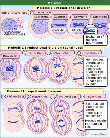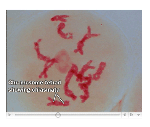Meiosis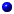Additional, physical evidence for these crossover events came much later, and were defined by Creighton and McClintock (1931) while trying to understand the gentic assortment of two genes in corn: Waxy [Wx] (starchy ) and Colour [C] (colourless). These two genes were found to be linked on chromosome #9, and their crossover phenotypes were always reflected in the physical aberrations at the extremities on each of the chromosomes.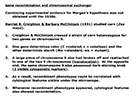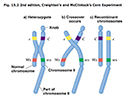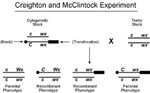Morgan went further.  He proposed that the frequency of such cross-overevents (occurring between two gene pairs) was a function of the genetic distance between the two loci..., BUT ONLY as the gene pairs were relatively quite close to each other on the chromosome.

He thus defined the unit of genetic distance as being:

one crossover event/100 products of meiosis = one map unit or 1 centiMorgan (cM)

If this were to be the case, then perhaps the apparent association or linkage could be used to define the order of the location of genes that were relatively close together onthe chromosome

The answer to this idea was... Yes, because the probability of crossing over occuring between two loci that were relatively close to each other on the same chromosome appeared to be additive.

Thus, in the following linkage map the probability of a crossing over event pccuring between Y and M would be expected to be 35%.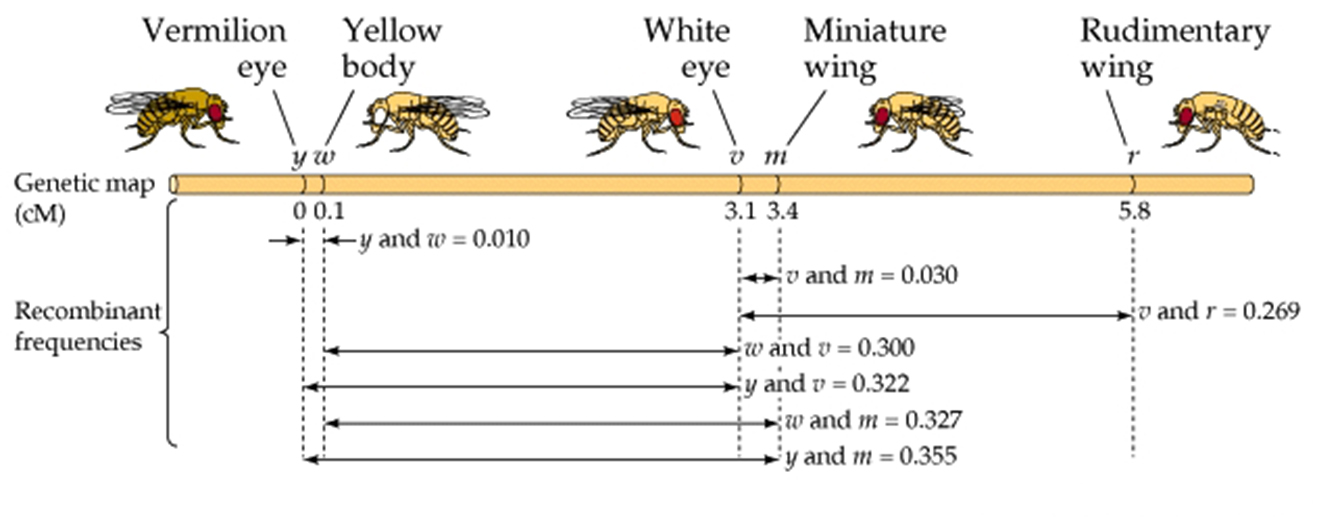Y-----W- -------------------------------------------M 0.5 34.5

One of Morgan's students, Sturtevant, quickly realized that such information could be used to map genes.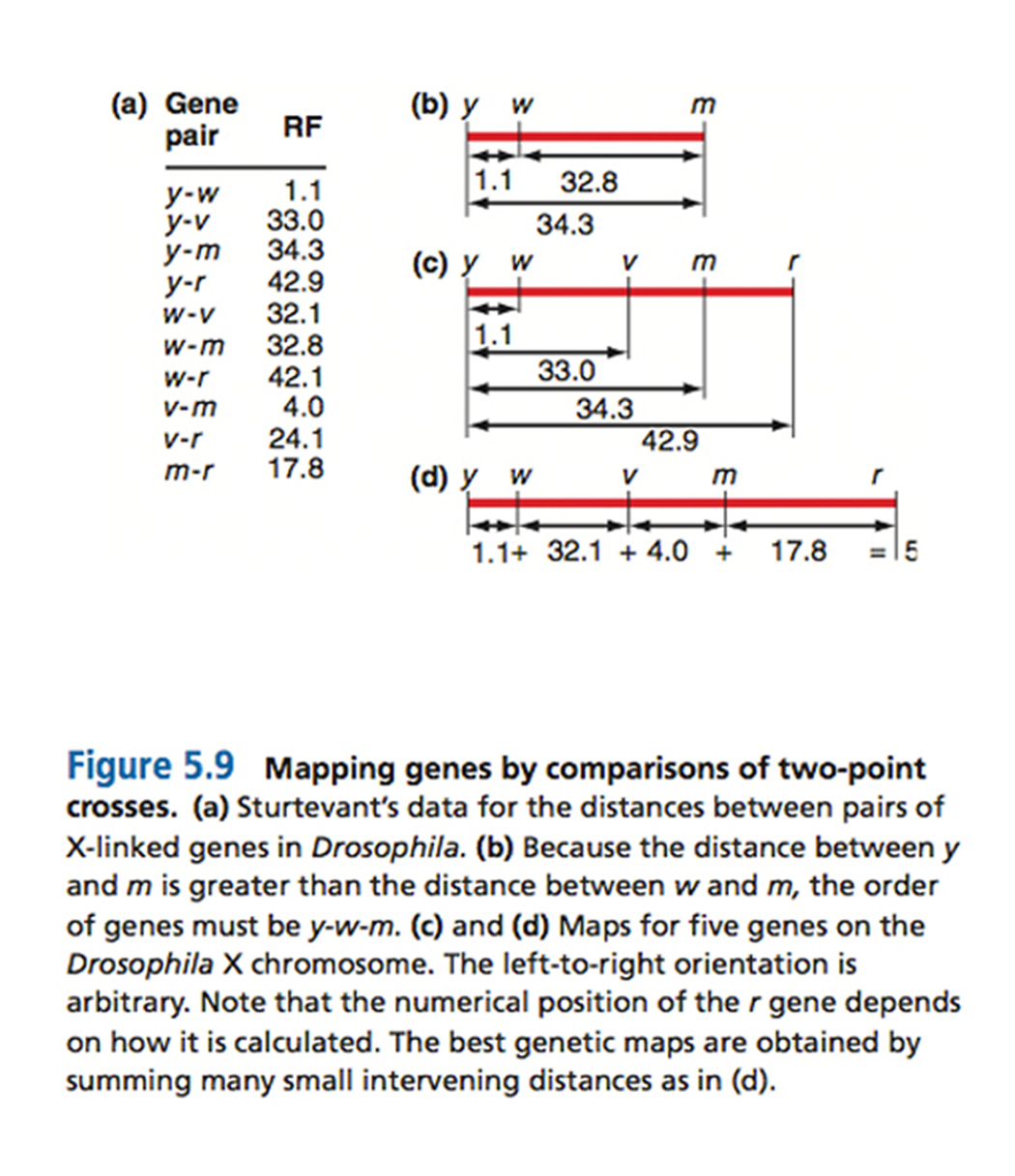Three point test crosses. Mathematically, two independent events happening at the same time-

However, to observe these traits three criteria had to be upheld...

1. Organism producing the crossover gametes must be heterozygous for all three traits.

2. The cross must be constructed so that each of the phenotypic traits must reflect a change in the genotype at each locus.

3. A sufficient number of offspring must be obtained to get statistically viable results.

In determining the gene order among three genes. You will need to know how this can be achieved!!

eg.

F1:

 a b c A B C

This is equivalent to the required heterozygotic parental generation for all three traits.

Remember that this is the simplest case where all the wild-type alleles are dominant and, at least in this case, on the same chromosome.  Correspondingly, ALL the mutant alleles are iniitially on the other.

In the next generation these triple heterozygotes can be test- crossed with triply recessive testers (???)............

In so doing, any "crossing-over" events that have occurred in the formation of the gametes will show through against the triply recessive pairing.

F2: listed as gametic genotypes...Crossover Types

 a b c = 230 = 467 = 42.1% = NCO A B C = 237 a B C = 82 = 161 = 14.5% = SCO A b c = 79 a b C = 200 = 395 = 35.6% = SCO A B c = 195 a B c = 44 = 86 = 7.8% = DCO A b C = 42

Thus, some vindication of the underlying linkage principle... but this all assumes the premise that the gene order is a b c

Another example: Cross of X/X y/y z/z with x/x Y/Y Z/Z is, however, not appear to behave is such an overtly simple manner, = -as there is now what is termed a mixed "association" of =Wild-Type and mutant alleles, giving:

F1

 X y z x Y Z

Now if this triply heterozygotic strain were to be three-point tested with a triple recessive...! yielding 1448 total offspring

 X y z = 580 = 1172 = 80.9% = NCO x Y Z = 592 X y Z = 45 = 85 = 5.9% = SCO x Y z = 40 X Y z = 89 = 183 = 12.6% = SCO x y Z = 94 X Y Z = 3 = 8 = 0.5% = DCO x y z = 5

But these problem only arise, however, if we assume the genes are in alphabetical order; that, with respect to x and y, the parent types are "X y z " and "x Y Z"

If they are not, then:

the First question would be....... What is the gene order?  We find this out by looking at the DCO's.

 X y z x Y Z

What would be the gene order that gives "X Y Z" as a DOUBLE cross over

The answer is determined empirically by trial and error...   "y     x      z".

Now both the single cross overs (SCO) and double crossovers DCO numbers make sense.

The reorganization of genes further allows us to guage the recombinant distance between the outside genes pairs of y and zby adding up the SCO values between y --- x and x --- z = 268 / 1448 =18.5% to be the recombination frequency value (RF) between the outside genes pairs of y and z

If one does the same thing for the other two sets of gene pairs then one can obtain recombination frequency values (RF values) of 13.1%  for y and x, and 6.4% for x and z, respectively

But 13.1 + 6.4 = 19.5%, which is a little higher than the previously calculated 18.5% -because the DCO's also need to be considered when evaluating crossover frequency.

The Second question: Are the relative rates of SCO's and DCO's consistent? If not... what is wrong?

Use of mathematical equation that was developed to predict the "true" probability of random events... Poisson distribution.

RF (recombination frequency) = 0.5 x (1 - e -m ).

which relates mapping units "m" (or the mean number of exchanges) directly to RF.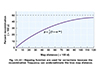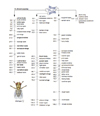-------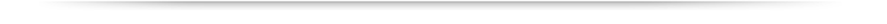Copyright © Department of Biology, Georgia State UniversityView Legal Statement Contact Biology Office: Tel: 404-413-5300
 About Academics Admissions Research Libraries About University Library Campus Life Athletics Alumni